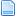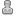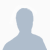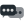Hello Guest it is September 19, 2020, 02:40:11 AM

###Author Topic: Machine pauses at Sub call  (Read 653 times)

0 Members and 1 Guest are viewing this topic.

####SinteredFuzz•28##### Machine pauses at Sub call
« on: November 14, 2017, 08:21:07 PM »
This code causes a pause/stop (about .25 seconds making it roughly 1/4th the time running)) at the commencement of each passing subroutine. It's killing the overall speed I can complete a pattern.
What's the cause? Can I do anything about it?
I'm not really experienced with the coding and thought this was a great idea but now have doubts.
Thanks Tom

Code below.

%
G20

#100= -0.001      (adapts Z depth out and back)
#102= -1.000      (adapts X points out)
#103=  1.000     (unused)
#104=  1.000      (adapts X points back)

F80

G01 Y1.000 (move to workpiece)
G01 X0.025421 Z0.020637
M98 P0099 L10     (LOOP# of overall passes, 1thou down and 1thou back)

O0099             (SUB resets length Vars, decrements depth)
#102= -1.000      (reset Var)
#103=  1.000      (reset Var)
#104=  1.000      (reset Var)
#100=[#100-0.001] (decrement 1thou)
M98 P0100 L72     (LOOP# determines repeats for X+ length)
#100=[#100-0.001] (decrement 1thou)
M98 P0101 L72     (LOOP# determines repeats for X- length and must match above)
M99

O0100         (SUB describes pattern out)
#102=[#102+1.000] (VAR adjusts for length of pattern each loop)

G02 X[0.025421+#102] Z[0.020637+#100] I-0.228622 J-0.352210
G02 X[0.048171+#102] Z[0.032509+#100] I-0.195165 J-0.346237
G02 X[0.071646+#102] Z[0.042863+#100] I-0.167154 J-0.347184
G02 X[0.095239+#102] Z[0.051403+#100] I-0.134389 J-0.334421
G02 X[0.119370+#102] Z[0.058249+#100] I-0.106942 J-0.331029
G02 X[0.143462+#102] Z[0.063192+#100] I-0.077110 J-0.314615
G02 X[0.167854+#102] Z[0.066259+#100] I-0.051180 J-0.308477
G02 X[0.192111+#102] Z[0.067335+#100] I-0.025102 J-0.291934
G02 X[0.213220+#102] Z[0.066468+#100] I-0.000708 J-0.240222
G02 X[0.232466+#102] Z[0.063867+#100] I-0.016724 J-0.196217
G02 X[0.251324+#102] Z[0.059320+#100] I-0.033499 J-0.180312
G02 X[0.269086+#102] Z[0.052942+#100] I-0.048794 J-0.163805
G02 X[0.286038+#102] Z[0.044647+#100] I-0.066699 J-0.157764
G02 X[0.302652+#102] Z[0.034155+#100] I-0.090535 J-0.161771
G02 X[0.318126+#102] Z[0.022008+#100] I-0.115476 J-0.163035
G02 X[0.333844+#102] Z[0.007100+#100] I-0.160726 J-0.185198
G02 X[0.356481+#102] Z[0.020660+#100] I-0.227297 J-0.353794
G02 X[0.379496+#102] Z[0.032529+#100] I-0.196095 J-0.351981
G02 X[0.403232+#102] Z[0.042874+#100] I-0.168550 J-0.354327
G02 X[0.427080+#102] Z[0.051409+#100] I-0.136139 J-0.342825
G02 X[0.451459+#102] Z[0.058255+#100] I-0.108441 J-0.339316
G02 X[0.475720+#102] Z[0.063193+#100] I-0.078012 J-0.321250
G02 X[0.500276+#102] Z[0.066263+#100] I-0.051686 J-0.313651
G02 X[0.524540+#102] Z[0.067335+#100] I-0.025112 J-0.293167
G02 X[0.545638+#102] Z[0.066463+#100] I-0.000703 J-0.238616
G02 X[0.564688+#102] Z[0.063865+#100] I-0.016439 J-0.191635
G02 X[0.583343+#102] Z[0.059310+#100] I-0.032884 J-0.175159
G02 X[0.600831+#102] Z[0.052935+#100] I-0.047955 J-0.158741
G02 X[0.617500+#102] Z[0.044645+#100] I-0.065832 J-0.153272
G02 X[0.633864+#102] Z[0.034147+#100] I-0.090666 J-0.159331
G02 X[0.641652+#102] Z[0.028246+#100] I-0.114204 J-0.158809
G02 X[0.649493+#102] Z[0.021681+#100] I-0.135048 J-0.169272
G02 X[0.657027+#102] Z[0.014762+#100] I-0.151485 J-0.172495
G02 X[0.664680+#102] Z[0.007100+#100] I-0.180969 J-0.188432
G02 X[0.687178+#102] Z[0.020694+#100] I-0.237613 J-0.367822
G02 X[0.709819+#102] Z[0.032536+#100] I-0.198470 J-0.351901
G02 X[0.733171+#102] Z[0.042890+#100] I-0.168690 J-0.348975
G02 X[0.756564+#102] Z[0.051415+#100] I-0.133884 J-0.331011
G02 X[0.780496+#102] Z[0.058253+#100] I-0.106150 J-0.326194
G02 X[0.804421+#102] Z[0.063195+#100] I-0.076353 J-0.309276
G02 X[0.828649+#102] Z[0.066256+#100] I-0.050711 J-0.303930
G02 X[0.852897+#102] Z[0.067335+#100] I-0.025088 J-0.290823
G02 X[0.874018+#102] Z[0.066473+#100] I-0.000712 J-0.241765
G02 X[0.893468+#102] Z[0.063868+#100] I-0.017034 J-0.201068
G02 X[0.912538+#102] Z[0.059328+#100] I-0.034141 J-0.185717
G02 X[0.930566+#102] Z[0.052949+#100] I-0.049569 J-0.168774
G02 X[0.947791+#102] Z[0.044650+#100] I-0.067374 J-0.161861
G02 X[0.964611+#102] Z[0.034180+#100] I-0.089792 J-0.162988
G02 X[0.980273+#102] Z[0.022020+#100] I-0.113336 J-0.162138
G02 X[0.995000+#102] Z[0.007000+#100] I-0.153445 J-0.178401
M99

O0101         (SUB describes pattern back)
#104=[#104-1.000] (VAR adjusts for length of pattern each loop)

G03 X[71.996021+#104] Z[0.007160+#100] I-0.153445 J-0.178401
G03 X[71.980273+#104] Z[0.022020+#100] I-0.113336 J-0.162138
G03 X[71.964611+#104] Z[0.034180+#100] I-0.089792 J-0.162988
G03 X[71.947791+#104] Z[0.044650+#100] I-0.067374 J-0.161861
G03 X[71.930566+#104] Z[0.052949+#100] I-0.049569 J-0.168774
G03 X[71.912538+#104] Z[0.059328+#100] I-0.034141 J-0.185717
G03 X[71.893468+#104] Z[0.063868+#100] I-0.017034 J-0.201068
G03 X[71.874018+#104] Z[0.066473+#100] I-0.000712 J-0.241765
G03 X[71.852897+#104] Z[0.067335+#100] I-0.025088 J-0.290823
G03 X[71.828649+#104] Z[0.066256+#100] I-0.050711 J-0.303930
G03 X[71.804421+#104] Z[0.063195+#100] I-0.076353 J-0.309276
G03 X[71.780496+#104] Z[0.058253+#100] I-0.106150 J-0.326194
G03 X[71.756564+#104] Z[0.051415+#100] I-0.133884 J-0.331011
G03 X[71.733171+#104] Z[0.042890+#100] I-0.168690 J-0.348975
G03 X[71.709819+#104] Z[0.032536+#100] I-0.198470 J-0.351901
G03 X[71.687178+#104] Z[0.020694+#100] I-0.237613 J-0.367822
G03 X[71.664680+#104] Z[0.007100+#100] I-0.180969 J-0.188432
G03 X[71.657027+#104] Z[0.014762+#100] I-0.151485 J-0.172495
G03 X[71.649493+#104] Z[0.021681+#100] I-0.135048 J-0.169272
G03 X[71.641652+#104] Z[0.028246+#100] I-0.114204 J-0.158809
G03 X[71.633864+#104] Z[0.034147+#100] I-0.090666 J-0.159331
G03 X[71.617500+#104] Z[0.044645+#100] I-0.065832 J-0.153272
G03 X[71.600831+#104] Z[0.052935+#100] I-0.047955 J-0.158741
G03 X[71.583343+#104] Z[0.059310+#100] I-0.032884 J-0.175159
G03 X[71.564688+#104] Z[0.063865+#100] I-0.016439 J-0.191635
G03 X[71.545638+#104] Z[0.066463+#100] I-0.000703 J-0.238616
G03 X[71.524540+#104] Z[0.067335+#100] I-0.025112 J-0.293167
G03 X[71.500276+#104] Z[0.066263+#100] I-0.051686 J-0.313651
G03 X[71.475720+#104] Z[0.063193+#100] I-0.078012 J-0.321250
G03 X[71.451459+#104] Z[0.058255+#100] I-0.108441 J-0.339316
G03 X[71.427080+#104] Z[0.051409+#100] I-0.136139 J-0.342825
G03 X[71.403232+#104] Z[0.042874+#100] I-0.168550 J-0.354327
G03 X[71.379496+#104] Z[0.032529+#100] I-0.196095 J-0.351981
G03 X[71.356481+#104] Z[0.020660+#100] I-0.227297 J-0.353794
G03 X[71.333844+#104] Z[0.007100+#100] I-0.160726 J-0.185198
G03 X[71.318126+#104] Z[0.022008+#100] I-0.115476 J-0.163035
G03 X[71.302652+#104] Z[0.034155+#100] I-0.090535 J-0.161771
G03 X[71.286038+#104] Z[0.044647+#100] I-0.066699 J-0.157764
G03 X[71.269086+#104] Z[0.052942+#100] I-0.048794 J-0.163805
G03 X[71.251324+#104] Z[0.059320+#100] I-0.033499 J-0.180312
G03 X[71.232466+#104] Z[0.063867+#100] I-0.016724 J-0.196217
G03 X[71.213220+#104] Z[0.066468+#100] I-0.000708 J-0.240222
G03 X[71.192111+#104] Z[0.067335+#100] I-0.025102 J-0.291934
G03 X[71.167854+#104] Z[0.066259+#100] I-0.051180 J-0.308477
G03 X[71.143462+#104] Z[0.063192+#100] I-0.077110 J-0.314615
G03 X[71.119370+#104] Z[0.058249+#100] I-0.106942 J-0.331029
G03 X[71.095239+#104] Z[0.051403+#100] I-0.134389 J-0.334421
G03 X[71.071646+#104] Z[0.042863+#100] I-0.167154 J-0.347184
G03 X[71.048171+#104] Z[0.032509+#100] I-0.195165 J-0.346237
G03 X[71.025000+#104] Z[0.020000+#100] I-0.228622 J-0.352210
M99
%